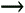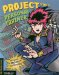# Lesson3.6.Understanding Effort-Driven Project Scheduling

### Lesson 3.6. Understanding Effort-Driven Project Scheduling

##### Figure 3-7. The scheduling formula is the basis for Project's scheduling calculations.Before diving into how to assign resources to tasks, it's important to understand the scheduling relationship between tasks and resources. Project schedules its tasks with effort-driven scheduling, which means that it tries to calculate the amount of effort, or work, required to complete a task. Project uses the scheduling formula, as shown in Figure 3-7, to do this.

Tip: You can turn off effort-driven scheduling for every new task that you create. Select ToolsSchedule tab, and uncheck the New tasks are effort driven checkbox.

Let's talk about how this formula is applied using an example. A task is scheduled to last 5 days, or 40 hours. When a resource is assigned to apply 100% of its working time on the task, the scheduling formula looks like this:

40 hours task duration x 100% resource units = 40 hours of work

Now Project knows that the task requires 40 hours of work to be completed.

Changing one of the variables in the formula affects another part of the formula. For example, what if you assigned another resource to work full-time on the task? The duration of the task would change:

20 hours task duration x 200% resource units = 40 hours of work

Thus, once assignments are created between tasks and resources, you as the project manager must understand how changing the variables of the scheduling formula affect other parts of the project.Project 2003 Personal Trainer
ISBN: 0596008546
EAN: 2147483647
Year: 2005
Pages: 156

Similar book on Amazon